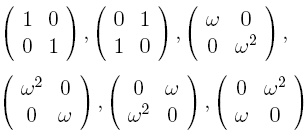occasional meanderings in physics' brave new world

## Friday, April 02, 2010

### M Theory Lesson 309

Recall that much of the tribimaximal mixing literature discusses small finite groups, such as $S_3$ and $A_4$. For example, Ernest Ma considers a $2 \times 2$ representation of the six element group given by the matriceswhere $\omega$ is the usual root of unity. This is an example of a fun set, with $d = 2$ and $n = 3$. In fact, it is the full such set under the condition that the non zero entries multiply to $1$. So special representations need no longer live in boring categories of vector spaces!Chess beater said...Kea said...